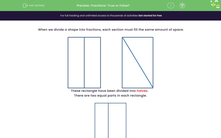# Recognise Fractions

In this worksheet, students will decide whether or not a shape shows a given fraction.Key stage:  KS 2

Curriculum topic:   Number: Fractions

Curriculum subtopic:   Use Fractions

Popular topics:   Fractions worksheets

Difficulty level:#### Worksheet Overview

When we divide a shape into fractions, each section must fill the same amount of space.These rectangles have been divided into halves.

There are two equal parts in each rectangle.This rectangle has not been divided into halves.

The two parts are not equal in area.Let's have a look at some questions now and see if we can recognise when a shape has been divided into halves.

### What is EdPlace?

We're your National Curriculum aligned online education content provider helping each child succeed in English, maths and science from year 1 to GCSE. With an EdPlace account you’ll be able to track and measure progress, helping each child achieve their best. We build confidence and attainment by personalising each child’s learning at a level that suits them.

Get started